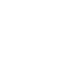# SAT Question of the Day (ACT too!): Mar. 26, 2014

If you are reading this in an email you received from me, do not click the link to sat.collegeboard.org below. Use the link to my website that is farther down on the email.

http://sat.collegeboard.org/practice/sat-question-of-the-day?questionId=20140326&oq=1 (This link takes you to today’s question. If you use my archive, you will see the question related to my SAT explanation for that date.)

The answer is E.  Yes, you should read the SAT staff’s explanation and if it makes sense to you, that would be a good way to do it.  However, there’s another, easier  way to handle this question for me.  Maybe it would be easier for you too.

Whenever I see a question about a line on the coordinate plane, I think “slope-intercept equation: y = mx + b.”  They tell me the line is “parallel to the x-axis” which easily translates into “no slope or a slope of 0.”  That means m has to be 0; so, there’s a 0x –or no x — in the equation.  And the y-intercept has to be 4 because the question tells us the line is “4 units above the x-axis.”  That leaves me with y = 0x + 4 or y = 4, Answer E.  That is a second way you could have done the math and gotten this question correct.

I did it a different way.  They tell you in the question that the line is always “parallel to the x– axis” and  “four units above the x-axis” which means y is always 4.  That has to be Answer E, y = 4.  Answers A and B are lines parallel to the y-axis. Answer C is a line that is parallel  to the x-axis but four units below it.  Answer D is the x-axis!  For me, this was approach was much faster than either of the first two.

My point today is that there is going to be more than one way to do the math questions.  You need to practice in order to determine the best ways to do the questions.  Keep in mind that both accuracy and speed are important.  For example, all three of these strategies got the right answer for today’s question, but I bet the third one is much faster for most students.

I wonder if the ACT folks have something new for us this morning.

This question is an example of what Ms. Murphy, my ninth grade English teacher, said a thousand times, “Shorter is better.”

The answer is C.  These two words make exactly the same point as the other options which range from three to seven words.  The other options even introduce some silly ideas.  For example, Answer D says the university was in the Midwest “at the time!”  What?  Did it get moved at a later time?  Highly doubtful.

Thought for the day:

Is winter ever going to go away?  We’ve been on a roller coaster weather pattern from winter to spring to winter again.  It reminds me of preparing for the SAT and ACT tests.  Your practice is going to involve some good days and some bad days.  Some days you’ll get almost all the questions correct and think you are going to do well.  The next day you could have a bad day and miss lots of questions.  Keep practicing until your performance is consistently at the level you need to succeed.  Hopefully, spring will get here and stay soon!

Enjoy the day.

Bob Alexander, the “SAT and ACT Wizard”